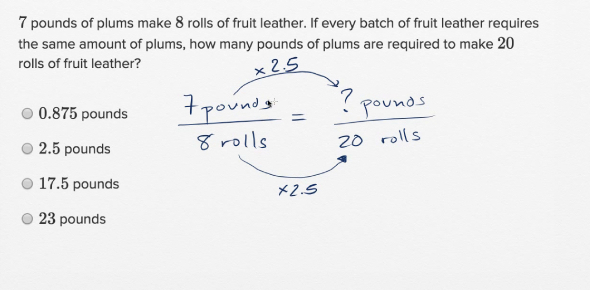# Practice Test Math Multiplication 2/1/08

10 Questions | Total Attempts: 284SettingsThis is a practice test on multiplication for Mrs. Currie's 6th grade class at Daybreak Middle School.

Related Topics
• 1.
Multiplication: 544 x 432 = Do work on a separate piece of paper. Do not use calculators.
• A.

236008

• B.

237008

• C.

235008

• D.

235908

• E.

234908

• 2.
Multiplation: 33 x 55 = Do work on a separate piece of paper. Do not use calculators.
• A.

1815

• B.

1715

• C.

1805

• D.

1705

• E.

1515

• 3.
Multiplation: 102 x 155 = Do work on a separate piece of paper. Do not use calculators.
• A.

25810

• B.

15800

• C.

15877

• D.

15810

• E.

15807

• 4.
Multiplation: 10,000 x 273 = Do work on a separate piece of paper. Do not use calculators.
• A.

273,000

• B.

2,730,000

• C.

27,300,000

• D.

3,730,000

• E.

373,000

• 5.
Multiplation: 1777 x 135 = Do work on a separate piece of paper. Do not use calculators.
• A.

238,985

• B.

237,895

• C.

237,995

• D.

239,895

• E.

240,985

• 6.
Multiplation: 32 x 23 Do work on a separate piece of paper. Do not use calculators.
• A.

636

• B.

726

• C.

736

• D.

626

• E.

756

• 7.
Multiplation: 200 x 45 = Do work on a separate piece of paper. Do not use calculators.
• A.

9000

• B.

4500

• C.

9500

• D.

9200

• E.

4900

• 8.
Multiplation: 237 x 50 = Do work on a separate piece of paper. Do not use calculators.
• A.

11150

• B.

11850

• C.

11150

• D.

10850

• E.

10150

• 9.
Multiplation: 991 x 47 = Do work on a separate piece of paper. Do not use calculators.
• A.

47977

• B.

46977

• C.

45577

• D.

42577

• E.

46577

• 10.
Multiplation: 555 x 303 = Do work on a separate piece of paper. Do not use calculators.
• A.

168,005

• B.

168,165

• C.

167,065

• D.

158,155

• E.

158,166